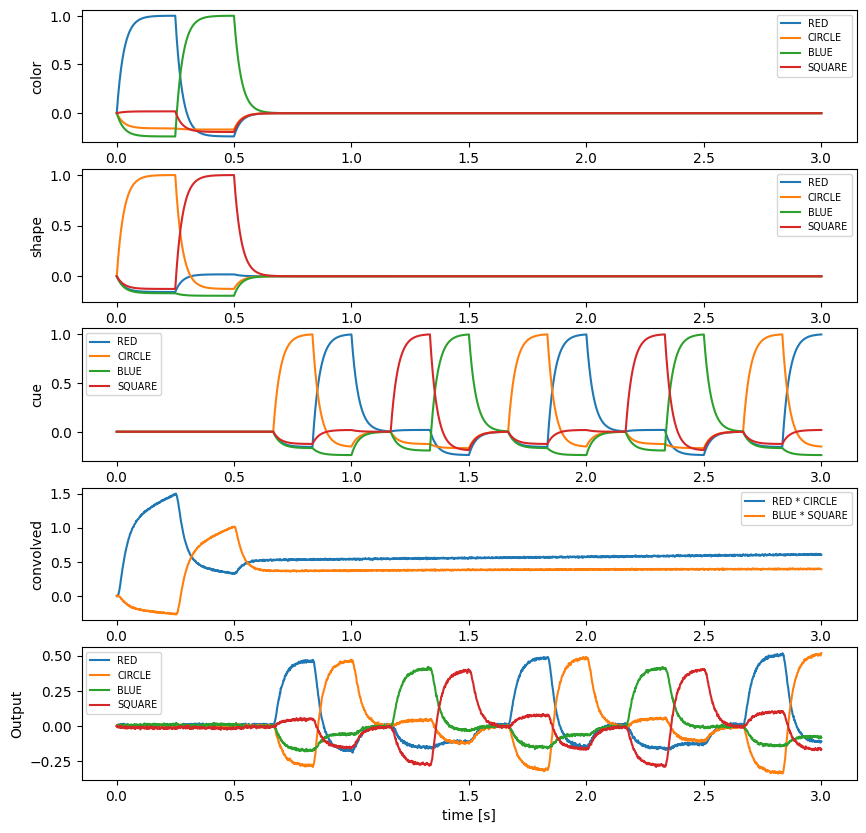Note

This documentation is for a development version. Click here for the latest stable release (v1.3.0).

# Simple question answering with memory¶

This demo implements a simple form of question answering. Two features (color and shape) will be bound by circular convolution and stored in a memory population. A cue will be used to determine either one of the features by deconvolution.

When you run the network, it will start by binding RED and CIRCLE for 0.25 seconds and then binding BLUE and SQUARE for 0.25 seconds. Both bound semantic pointers are stored in a memory population. Then the network is asked with the cue. For example, when the cue is CIRCLE the network will respond with RED.

:

%matplotlib inline

import nengo
import matplotlib.pyplot as plt

import nengo_spa as spa

seed = 0


## Define the input functions¶

The color input will RED and then BLUE for 0.25 seconds each before being turned off. In the same way the shape input will be CIRCLE and then SQUARE for 0.25 seconds each. Thus, the network will bind alternatingly RED * CIRCLE and BLUE * SQUARE for 0.5 seconds each.

The cue for deconvolving bound semantic pointers will be turned off for 0.5 seconds and then cycles through CIRCLE, RED, SQUARE, and BLUE within one second.

:

def color_input(t):
if t < 0.25:
return "RED"
elif t < 0.5:
return "BLUE"
else:
return "0"

def shape_input(t):
if t < 0.25:
return "CIRCLE"
elif t < 0.5:
return "SQUARE"
else:
return "0"

def cue_input(t):
if t < 0.5:
return "0"
sequence = ["0", "CIRCLE", "RED", "0", "SQUARE", "BLUE"]
idx = int(((t - 0.5) // (1.0 / len(sequence))) % len(sequence))
return sequence[idx]


## Create the model¶

:

# Number of dimensions for the Semantic Pointers
dimensions = 32

model = spa.Network(label="Simple question answering", seed=seed)

with model:
color_in = spa.Transcode(color_input, output_vocab=dimensions)
shape_in = spa.Transcode(shape_input, output_vocab=dimensions)
conv = spa.State(dimensions, subdimensions=4, feedback=1.0, feedback_synapse=0.4)
cue = spa.Transcode(cue_input, output_vocab=dimensions)
out = spa.State(dimensions)

# Connect the buffers
color_in * shape_in >> conv
conv * ~cue >> out


## Probe the output¶

:

with model:
model.config[nengo.Probe].synapse = nengo.Lowpass(0.03)
p_color_in = nengo.Probe(color_in.output)
p_shape_in = nengo.Probe(shape_in.output)
p_cue = nengo.Probe(cue.output)
p_conv = nengo.Probe(conv.output)
p_out = nengo.Probe(out.output)


## Run the model¶

:

with nengo.Simulator(model) as sim:
sim.run(3.0)


## Plot the results¶

:

plt.figure(figsize=(10, 10))
vocab = model.vocabs[dimensions]

plt.subplot(5, 1, 1)
plt.plot(sim.trange(), spa.similarity(sim.data[p_color_in], vocab))
plt.legend(vocab.keys(), fontsize="x-small")
plt.ylabel("color")

plt.subplot(5, 1, 2)
plt.plot(sim.trange(), spa.similarity(sim.data[p_shape_in], vocab))
plt.legend(vocab.keys(), fontsize="x-small")
plt.ylabel("shape")

plt.subplot(5, 1, 3)
plt.plot(sim.trange(), spa.similarity(sim.data[p_cue], vocab))
plt.legend(vocab.keys(), fontsize="x-small")
plt.ylabel("cue")

plt.subplot(5, 1, 4)
for pointer in ["RED * CIRCLE", "BLUE * SQUARE"]:
plt.plot(sim.trange(), vocab.parse(pointer).dot(sim.data[p_conv].T), label=pointer)
plt.legend(fontsize="x-small")
plt.ylabel("convolved")

plt.subplot(5, 1, 5)
plt.plot(sim.trange(), spa.similarity(sim.data[p_out], vocab))
plt.legend(vocab.keys(), fontsize="x-small")
plt.ylabel("Output")
plt.xlabel("time [s]")

:

Text(0.5, 0, 'time [s]')The plots of shape, color, and convolved show that first RED * CIRCLE and then BLUE * SQUARE will be loaded into the convolved buffer so after 0.5 seconds it represents the superposition RED * CIRCLE + BLUE * SQUARE.

The last plot shows that the output is most similar to the semantic pointer bound to the current cue. For example, when RED and CIRCLE are being convolved and the cue is CIRCLE, the output is most similar to RED. Thus, it is possible to unbind semantic pointers from the superposition stored in convolved.